Question

A store sells bags of candy that weigh exactly 2lb, according to their packaging. Elena, the store manager tests the claim by hypothesizing that the population mean weight of the bags is not equal to 2lb. She randomly selects 15 bags and found that the sample mean weight is 1.9968lb with a standard deviation of 0.0286lb. Assuming the weights of the bags are normally distributed, the test statistic t for a hypothesis test of H0:μ=2 versus Ha:μ≠2 is t≈−0.43 with 14degrees of freedom. If p-value>0.20 and the level of significance is α=0.05, which of the following statements are accurate for this hypothesis test to evaluate the claim that the true population mean weight of the bags is significantly not equal to 2lb?

Select all that apply:

A) Reject the null hypothesis that the true population mean weight of the bags is equal to 2lb.

B) Fail to reject the null hypothesis that the true population mean weight of the bags is equal to 2lb.

C) There is enough evidence at the α=0.05 level of significance to support the claim that the true population mean weight of the bags is not equal to 2lb.

D) There is not enough evidence at the α=0.05 level of significance to suggest that the true population mean weight of the bags is not equal to 2lb.
Expert Solution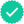Students who’ve seen this question also like: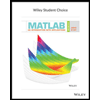Your question is solved by a Subject Matter ExpertWant to see this answer and more?
Experts are waiting 24/7 to provide step-by-step solutions in as fast as 30 minutes!*
*Response times may vary by subject and question complexity. Median response time is 34 minutes for paid subscribers and may be longer for promotional offers.8+ million solutionsSupport from experts
Send experts your homework questions or start a chat with a tutorEssay support
Check for plagiarism and create citations in secondsSolve math equations
Get instant explanations to difficult math equations
Students love us.
Students love us.
Knowledge BoosterRecommended textbooks for you
•MATLAB: An Introduction with Applications
Statistics
ISBN:9781119256830
Author:Amos Gilat
Publisher:John Wiley & Sons Inc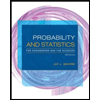Probability and Statistics for Engineering and th...
Statistics
ISBN:9781305251809
Author:Jay L. Devore
Publisher:Cengage Learning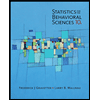Statistics for The Behavioral Sciences (MindTap C...
Statistics
ISBN:9781305504912
Author:Frederick J Gravetter, Larry B. Wallnau
Publisher:Cengage Learning
•Elementary Statistics: Picturing the World (7th E...
Statistics
ISBN:9780134683416
Author:Ron Larson, Betsy Farber
Publisher:PEARSON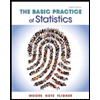The Basic Practice of Statistics
Statistics
ISBN:9781319042578
Author:David S. Moore, William I. Notz, Michael A. Fligner
Publisher:W. H. Freeman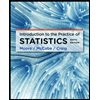Introduction to the Practice of Statistics
Statistics
ISBN:9781319013387
Author:David S. Moore, George P. McCabe, Bruce A. Craig
Publisher:W. H. Freeman
•MATLAB: An Introduction with Applications
Statistics
ISBN:9781119256830
Author:Amos Gilat
Publisher:John Wiley & Sons IncProbability and Statistics for Engineering and th...
Statistics
ISBN:9781305251809
Author:Jay L. Devore
Publisher:Cengage LearningStatistics for The Behavioral Sciences (MindTap C...
Statistics
ISBN:9781305504912
Author:Frederick J Gravetter, Larry B. Wallnau
Publisher:Cengage LearningElementary Statistics: Picturing the World (7th E...
Statistics
ISBN:9780134683416
Author:Ron Larson, Betsy Farber
Publisher:PEARSONThe Basic Practice of Statistics
Statistics
ISBN:9781319042578
Author:David S. Moore, William I. Notz, Michael A. Fligner
Publisher:W. H. FreemanIntroduction to the Practice of Statistics
Statistics
ISBN:9781319013387
Author:David S. Moore, George P. McCabe, Bruce A. Craig
Publisher:W. H. Freeman